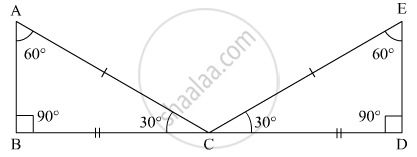Advertisement Remove all ads

# In Triangles Abc and Cde, If Ac = Ce, Bc = Cd, ∠A = 60°, ∠C = 30° and ∠D = 90°. Are Two Triangles Congruent? - Mathematics

Answer in Brief

In triangles ABC and CDE, if AC = CE, BC = CD, ∠A = 60°, ∠C = 30° and ∠D = 90°. Are two triangles congruent?

Advertisement Remove all ads

#### Solution

For the triangles ABC and ECD, we have the following information and corresponding figure:

AC = CE

BC = CD

∠A = 60°

∠C = 30°

∠D = 90°In triangles ABC and ECD, we have

AC = EC

BC = CD

and ∠BAC= ∠CED

The SSA criteria for two triangles to be congruent are being followed. So both the triangles are congruent.

Concept: Criteria for Congruence of Triangles
Is there an error in this question or solution?
Advertisement Remove all ads

#### APPEARS IN

RD Sharma Mathematics for Class 9
Chapter 12 Congruent Triangles
Q 5 | Page 84
Advertisement Remove all ads
Advertisement Remove all ads
Share
Notifications

View all notifications

Forgot password?
Course